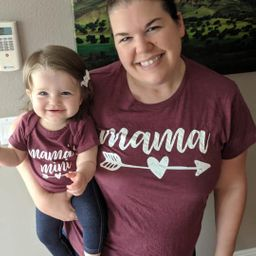Ask your homework questions to teachers and professors, meet other students, and be entered to win $600 or an Xbox Series X 🎉Join our Discord!Numerade Educator ### Problem 62 Hard Difficulty # The following table contains the test grades for the students in a chemistry class. Find the mean, median, and mode.$$\begin{array}{l|c|l|c}\text { Student } & \text { Score } & \text { Student } & \text { Score } \\ \hline \text { Allen, G. } & 84 & \text { Harris, A. } & 76 \\ \hline \text { Baker, S. } & 75 & \text { Litle, M. } & 71 \\ \hline \text { Barren, T. } & 64 & \text { Moore, S. } & 93 \\ \hline \text { Carter, J. } & 93 & \text { Payne, J. } & 60 \\ \hline \text { Donaldson, R. } & 82 & \text { Reiche, D. } & 78 \\ \hline \text { Green, R. } & 60 & \text { Rizvi, Z. } & 100 \\ \hline\end{array}$$ ### Answer ## mean score$=78$, median score$=77$and mode score$=60\$ and 93

#### Topics

No Related Subtopics

### Discussion

You must be signed in to discuss.

### Video Transcript

so write out all over, immersed in numerical order and start off to find the Me and I added all of these numbers together. When we do that, we get in 1936 and then divide by the number of items on our list for just 12. That leaves us with 78. Next, we'll find the mode. The mode is the number that occurs the most number of times you can see that 60 occurs twice, as does 93. So 60 and that 93 are both considered our mode. Finally, we want to find the median by finding the numbers in the middle. Since we have an even number of items will find the two numbers in the middle, 76 78 and we'll add those together and divide by two. When we do that, we get 77Other Schools

#### Topics

No Related Subtopics